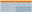Practice Numerical Estimation Tests

# Free Practice Numerical Estimation Tests

Updated October 26, 2021

## What Is Numerical Estimation?

Numerical estimation is key in many craft and technical jobs where the ability to quickly and accurately estimate material quantities is essential.

Numerical estimation questions usually form only part of a numerical ability test and you can also expect numerical computation questions.Numerical Estimation Tests

This sample question paper contains 40 questions and has a suggested time limit of 10 minutes. The questions are presented in Letter/A4 format for easy printing and self-marking.

Even though numerical estimation questions appear straightforward, it can take some time to develop the optimum compromise between speed and accuracy.

Before you attempt to answer each question, look at the range of answers available and ask yourself how accurate your estimate needs to be.

For example, is an order of magnitude sufficient or does the answer need to be worked out to the nearest whole number?

If you out of practice with arithmetic, then try re-learning the times tables up to 12 and practice rough and ready multiplication, division and percentage calculations.

Practice can improve your test scores for all types of aptitude tests but numerical estimation is one area where it can really make a difference, so try as many examples as you can.

These sample numerical estimation questions are directly applicable to tests used to select for craft and technical jobs.

However, the ability to make quick estimates is a useful skill to have even if you are sitting a graduate or professional level test as it will enable you to roughly check your answers to data interpretation questions.